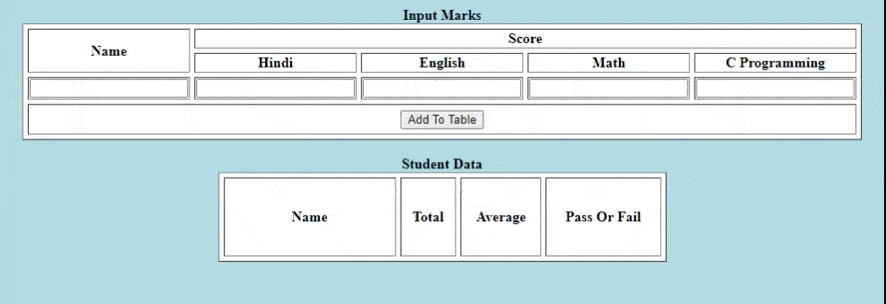# How to create a dynamic report card using HTML CSS and JavaScript ?

• Last Updated : 03 Aug, 2021

We have to build a website where you can upload your student data like their name, marks in different subjects. And after uploading it will insert the student data in the table as well as, it will show the total marks, average, and pass/fail status. The implementation is done by using HTML and JavaScript.

Approach:

Hey geek! The constant emerging technologies in the world of web development always keeps the excitement for this subject through the roof. But before you tackle the big projects, we suggest you start by learning the basics. Kickstart your web development journey by learning JS concepts with our JavaScript Course. Now at it's lowest price ever!

• At first, we have to create some rows and columns for name and subject scores. Again subject scores will be further divided into four columns. There are four subject names that will be displayed. Users are allowed to enter the details with a click button to “Add To Table”.
• There will be another table Student Data with four columns Name, Total, Average, Pass Or Fail.
• For every entered data, one row will add to the score table, showing the student’s total score, average and pass or fail status. If the average score is greater than 70 then the status is “pass” otherwise the status is “fail”.
• If we enter another data it will store in the table.

Example:

## HTML

 ```<``html``>`` ` `<``body` `bgcolor``=``"lightblue"``>``    ``<``center``>``    ``<``table` `border``=``"1"` `cellspacing``=``"5"` `bgcolor``=``"white"``>``        ``<``caption``><``b``>Input Marks``        ``<``tr``>``            ``<``th` `rowspan``=``"2"``>Name``            ``<``th` `colspan``=``"4"``>Score`` ` `        ````        ``<``tr``>``            ``<``th``>Hindi``            ``<``th``>English``            ``<``th``>Math``            ``<``th``>C Programming``        ````        ``<``tr``>``            ``<``td``><``input` `type``=``"text"` `id``=``"aname"``>``            ``<``td``><``input` `type``=``"text"` `id``=``"am"``>``            ``<``td``><``input` `type``=``"text"` `id``=``"aj"``>``            ``<``td``><``input` `type``=``"text"` `id``=``"ad"``>``            ``<``td``><``input` `type``=``"text"` `id``=``"an"``>``        ````        ``<``tr``>``            ``<``th` `colspan``=``"5"` `height``=``"30"``>``            ``<``input` `type``=``"submit"` `value``=``"Add To Table"` `onclick``=``"Sub()"``>``        ``    ``    ````    ``<``br``>``    ``<``table` `border``=``"1"` `cellspacing``=``"5"` `bgcolor``=``"white"` `           ``height``=``"100"` `width``=``"500"` `cellpadding``=``"5"` `id``=``"TableScore"``>``        ``<``caption``><``b``>Student Data``        ``<``tr``>``            ``<``th` `width``=``"180"``>Name``            ``<``th``>Total``            ``<``th``>Average``            ``<``th``>Pass Or Fail``        ````         ` `    ````    ````    ``<``script` `type``=``"text/javascript"``>``        ``function Sub(){``            ``var n, k, r, e, v, sum, avg;``            ``n=(document.getElementById('aname').value);``            ``k=parseFloat(document.getElementById('am').value);``            ``r=parseFloat(document.getElementById('aj').value);``            ``e=parseFloat(document.getElementById('ad').value);``            ``v=parseFloat(document.getElementById('an').value);``            ``// Calculating Total``            ``sum=k+r+e+v;``            ``avg=sum/4;``            ``// Display on Student Data``            ``var newTable = document.getElementById('TableScore');``            ``var row = newTable.insertRow(-1);``            ``var cell1 = row.insertCell(0);``            ``var cell2 = row.insertCell(0);``            ``var cell3 = row.insertCell(0);``            ``var cell4 = row.insertCell(0);`` ` `            ``cell4.innerHTML= n;``            ``cell3.innerHTML=sum;``            ``cell2.innerHTML = avg;`` ` `            ``if(avg>=70){``                ``cell1.innerHTML="<``font` `color``=``green``>Pass";``            ``}else{``                ``cell1.innerHTML="<``font` `color``=``red``>Fail";``            ``}``        ``}``    ```````

Output:Pass or Fail status of Students

My Personal Notes arrow_drop_up